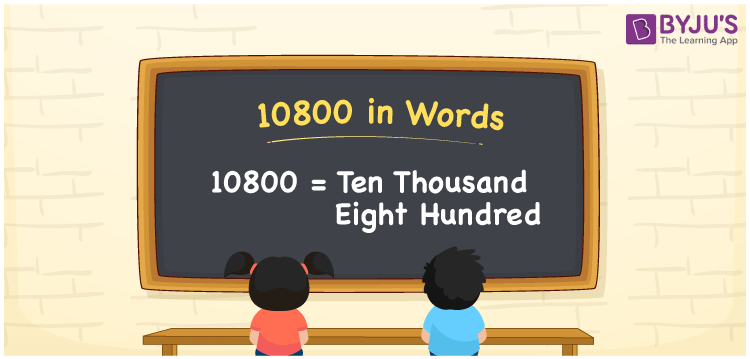# 10800 in Words

10800 in words is written as “Ten thousand eight hundred”. In Maths, 10800 is a natural number, that is used to represent a value or a count. Hence, 10800 is given by Ten thousand eight hundred in English. In real life also, we use numbers in words to express a value. For example, Rs.10800 is expressed as “Rupees Ten thousand eight hundred only” in a cheque.

 10800 in Words Ten thousand eight hundred Ten thousand eight hundred in numerals 10800

## 10800 in English Words## How to Write 10800 in Words?

We can convert 10800 to words using a place value chart, that represents the positions of digits in a number. The number 10800 has 5 digits, hence, the digits are represented as

 Ten thousand Thousands Hundreds Tens Ones 1 0 8 0 0

We can see, from the above table,

1 → Ten Thousands

0 → Thousands

8 → Hundreds

0 → Tens

0 → Ones

Now, read the digits from right to left. Thus, together we can write the number as Ten thousand eight hundred.

### Expanded Form of 10800

We can write the expanded form as:

1 × Ten thousand + 0 × Thousand + 8 × Hundred + 0 × Ten + 0 × One

= 1 × 10000 + 0 × 1000 + 8 × 100 + 0 × 10 + 0 × 1

= 10800

= Ten thousand eight hundred

10800 is the natural number that is succeeded by 10799 and preceded by 10801. Learn more about the number 10800 below:

• 10800 in Words – Ten thousand eight hundred
• Is 10800 an odd number? – No
• Is 10800 an even number? – Yes
• Is 10800 a perfect square number? – No
• Is 10800 a perfect cube number? – No
• Is 10800 a prime number? – No
• Is 10800 a composite number? – Yes

## Frequently Asked Questions on 10800 in words

Q1

### What is 10800 in words?

10800 in words is given by Ten thousand eight hundred.
Q2

### What is the place value of 8  in 10800?

The place value of 8 in 10800 is Hundreds.
Q3

### What is 10800 plus 2000 in words?

10800 + 2000 = 12800, i.e., Twelve thousand eight hundred.
Test your Knowledge on 10800 in Words